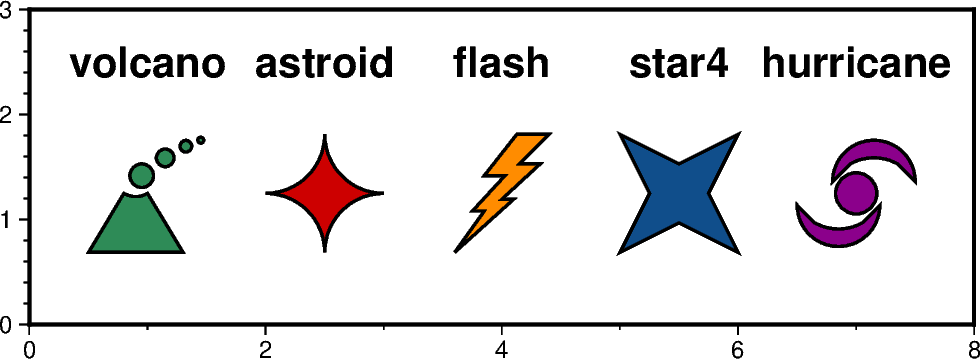# Custom symbols

The `pygmt.Figure.plot` method can plot individual custom symbols by passing the corresponding symbol name together with the k shortcut to the `style` parameter. In total 41 custom symbols are already included of which the following plot shows five exemplary ones. The symbols are shown underneath their corresponding names. For the remaining symbols see the GMT cookbook https://docs.generic-mapping-tools.org/latest/cookbook/custom-symbols.html.```import pygmt

fig = pygmt.Figure()
fig.basemap(region=[0, 8, 0, 3], projection="X12c/4c", frame=True)

# define pen and fontstyle for annotations
pen = "1p,black"
font = "15p,Helvetica-Bold"

# use the volcano symbol with a size of 1.5c,
# fill color is set to "seagreen"
fig.plot(x=1, y=1.25, style="kvolcano/1.5c", pen=pen, fill="seagreen")
fig.text(x=1, y=2.5, text="volcano", font=font)

# use the astroid symbol with a size of 1.5c,
# fill color is set to "red3"
fig.plot(x=2.5, y=1.25, style="kastroid/1.5c", pen=pen, fill="red3")
fig.text(x=2.5, y=2.5, text="astroid", font=font)

# use the flash symbol with a size of 1.5c,
# fill color is set to "darkorange"
fig.plot(x=4, y=1.25, style="kflash/1.5c", pen=pen, fill="darkorange")
fig.text(x=4, y=2.5, text="flash", font=font)

# use the star4 symbol with a size of 1.5c,
# fill color is set to "dodgerblue4"
fig.plot(x=5.5, y=1.25, style="kstar4/1.5c", pen=pen, fill="dodgerblue4")
fig.text(x=5.5, y=2.5, text="star4", font=font)

# use the hurricane symbol with a size of 1.5c,
# fill color is set to "magenta4"
fig.plot(x=7, y=1.25, style="khurricane/1.5c", pen=pen, fill="magenta4")
fig.text(x=7, y=2.5, text="hurricane", font=font)

fig.show()
```

Total running time of the script: ( 0 minutes 1.381 seconds)

Gallery generated by Sphinx-Gallery